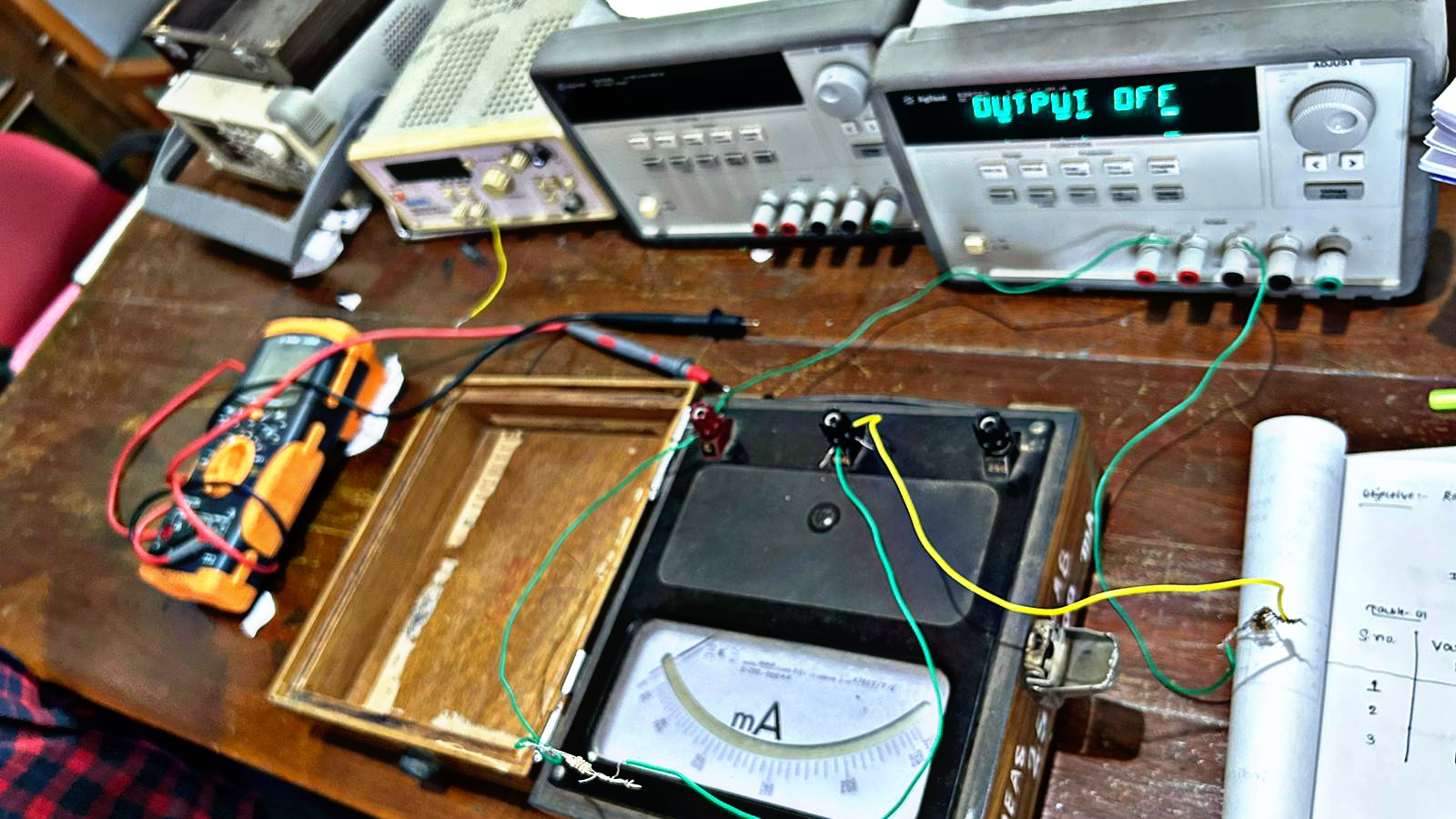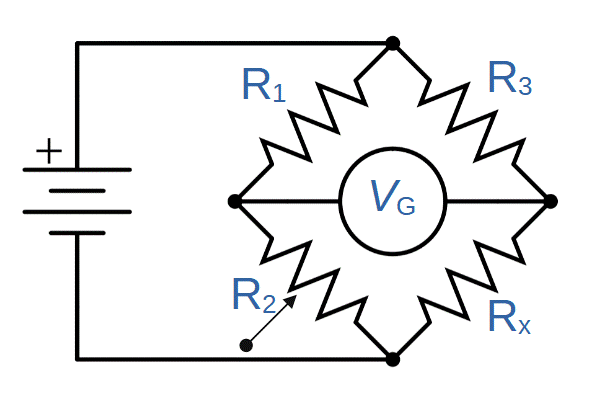# Unveiling Hidden Resistance: Unearthing the Unknown Resistor using the Wheatstone BridgeThere are lots of methods for finding the value of unknown resistance one of them is from the whetstone bridge.
We can find the value of unknown resistance is by the help of color coding but if we are unable to see properly the colors of resistance so we can use the bridge method to find the unknown resistance mostly this method is used to find the balance resistance of the circuit because to find the balance resistance of the circuit is difficult. Balance resistance means if you want to calculate the whole resistance of a circuit but there is a complex circuit available so to find the whole resistance of that circuit is much more difficult so this method is easier to calculate the resistance of that circuit.### Components are required to apply the bridge method

To apply this method we need to require three known resistance which has finite value and a galvanometer a DC power source that produces a voltage not more than 15 volts and there should be present a variable register.

### Galvanometer

It's a very sensitive instrument it measures the value of current but that's what I am says previously it is a very sensitive instrument so we can measure very very minimum value. But this instrument is not giving us the exact value of the current it's so only fluctuation that indicates the current is flowing there are lots of methods in which we can convert a galvanometer into a meter and voltmeter also we are not going to Deep in that.

### DC Power Source

For this method, we required a DC power source also but for this power source we need to require only 15-volt value research this method is applied only for the medium range of resistance not too much less not too much high.

### Variable Register

That should be presently available register in this method because we want to balance this circuit so for balancing the circuit we required a variable Register.

### Principal of Wheatstone Bridge

If four Registers are connected in a bridge and the power supply is applied on two terminals and the second both terminal is connected with a galvanometer if the galvanometer has zero deflection so this circuit is balanced.
Then the ratio of two registers is equal to the ratio of another two registers.

### Circuit Diagramsrc

### Formula Usedsrc

### Limitations of this method

The limitations of this method are we can use this method only for the medium range of resistance we cannot use this method for high and low values of resistance.

### Application

This method, we can use this to measure parameters like impedance, inductance, and capacitance resistance.

Posted with STEMGeeks

0
0
0.000Thanks for your contribution to the STEMsocial community. Feel free to join us on discord to get to know the rest of us!

Please consider delegating to the @stemsocial account (85% of the curation rewards are returned).

You may also include @stemsocial as a beneficiary of the rewards of this post to get a stronger support.

0
0
0.000I love your explanation of this circuit. I think I'll stick to reading the color of the bands for now.

0
0
0.000